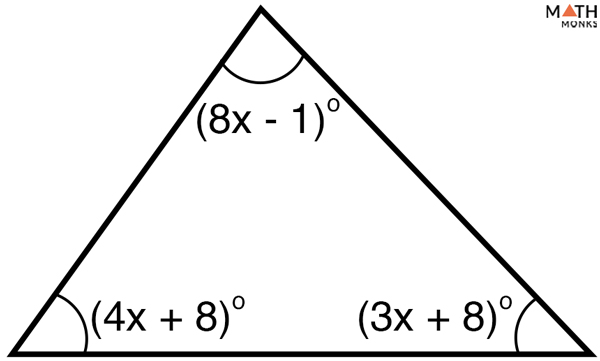# Triangle Sum Theorem

## What is the Triangle Sum Theorem

The triangle sum theorem states that the sum of the three interior angles in a triangle adds up to 180°. It is also called the angle sum theorem.

Given below is a triangle ABC, having three interior angles ∠a, ∠b, and ∠c. According to the triangle sum theorem, ∠a + ∠b + ∠c = 180°

## Prove Triangle Sum Theorem

To prove:

∠CBA + ∠BAC + ∠ACB = 180°

Proof:

A line PQ is drawn parallel to BC passing through the point A

Hence proved that, the sum of the three interior angles in a triangle adds up to 180°.

## Solved Examples

Let us solve some problems to understand the theorem better.Find the value of the unknown angle of the given triangle.

Solution:

As we know, according to the triangle sum theorem,
x + 38° + 32° = 180°
=> x = 180° – (38° + 32°)
=> x = 110°

Two interior angles of a triangle measure 30° and 80°. What is the third interior angle of the triangle?

Solution:

Let the third interior angle be x
As we know, according to the triangle sum theorem,
x + 30° + 80° = 180°
x = 180° – (30° + 80°)
x = 70°Solve the value of x and the measure of each angle.

Solution:

As we know, according to the triangle sum theorem,
(8x – 1)° + (4x + 8)° + (3x + 8)° = 180°
15x + 15 = 180°
15x = 165
x = 11°

## Corollary to the Triangle Sum Theorem

Two corollaries to the triangle sum theorem are:

Corollary 1: The acute angles of a right triangle are complementary (add up to 90°)

Hypothesis: From the triangle sum theorem, the sum of all three angles equals 180°

Again, from the definition of a right triangle, we have one of its angles to be a right angle, making the remaining angles to be acute whose sum equals (180° – 90°) is 90°

Conclusion: The acute angles of a right triangle are complementary

Corollary 2: Each angle in an equilateral triangle measures 60°

Hypothesis: From the triangle sum theorem, the sum of all three angles equals 180°

Again, from the definition of an equilateral triangle, all angles are of equal measure. Adding up all the angles, we get,

⇒ x + x + x = 180°

⇒ 3x = 180°

⇒ x = 60°

Conclusion: Each angle in an equilateral triangle measures 60°